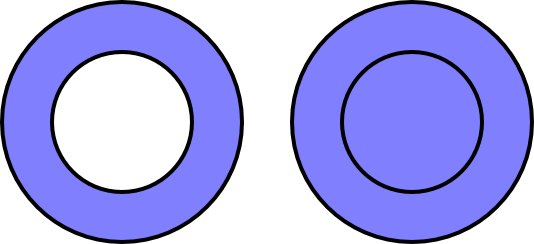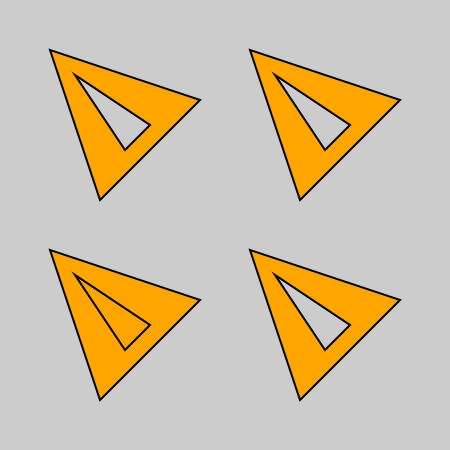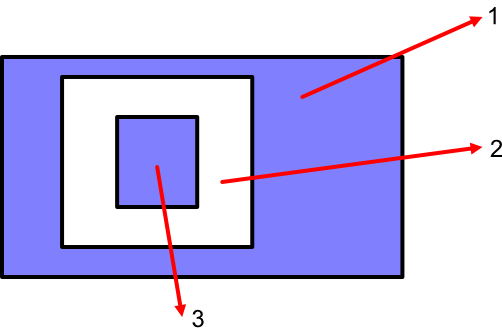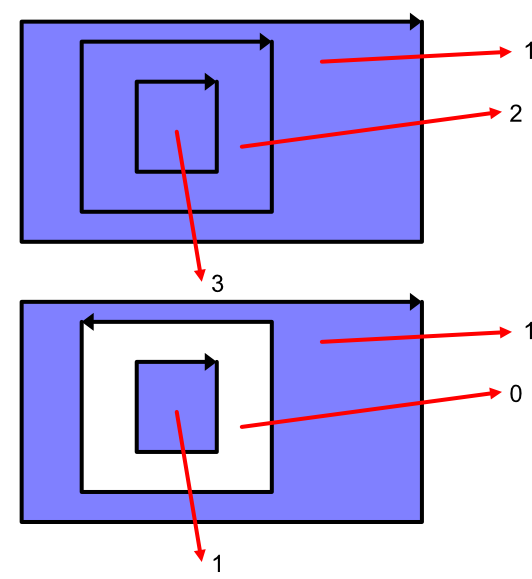# Fill styles in generativepy

Martin McBride, 2021-12-12
Tags generativepy tutorial fill stroke rectangle
Categories generativepy generativepy tutorialFilling a simple shape is easy, we just fill the entire shape. When we draw complex shapes, it can get a bit more complicated because we have to choose which parts of the shape should be filled.

This section only applies to complex paths, that is a path that is either self-intersecting or contains two or more shapes that are part of a single path

For example, a doughnut shape (or torus to give it its correct name) is a single path formed from two concentric circles. When we fill if, do we fill the entire shape, or leave a hole?By default, the outer shape gets filled. Any shapes inside that (provided they are part of the same complex path) will be left as holes. If that is what you want to do, you don't need to worry too much about this section because that is what will happen by default.

But sometimes we might like to have the choice, especially when it comes to even more complex shapes.

The fill method provides two options:

• EVEN_ODD, the default, simply cuts holes (and fills any holes in the holes).
• WINDING is a bit more complicated, but it gives you the option to control the filled areas much more selectively.

You should read the main fill and stroke article before this one.

## Example code

Here is a sample Python program for creating lines with various line end and dash styles:

from generativepy.drawing import make_image, setup, EVEN_ODD, WINDING
from generativepy.color import Color
from generativepy.geometry import Triangle

def draw4(ctx, pixel_width, pixel_height, frame_no, frame_count):
setup(ctx, pixel_width, pixel_height, background=Color(0.8))

black = Color(0)
orange = Color('orange')

Triangle(ctx).of_corners((50, 50), (200, 100), (100, 200)).add()
Triangle(ctx).of_corners((75, 75), (150, 125), (125, 150)).as_sub_path()\
.fill(orange, fill_rule=EVEN_ODD).stroke(black, 2)
Triangle(ctx).of_corners((250, 50), (400, 100), (300, 200)).add()
Triangle(ctx).of_corners((275, 75), (325, 150), (350, 125)).as_sub_path()\
.fill(orange, fill_rule=EVEN_ODD).stroke(black, 2)

Triangle(ctx).of_corners((50, 250), (200, 300), (100, 400)).add()
Triangle(ctx).of_corners((75, 275), (150, 325), (125, 350)).as_sub_path()\
.fill(orange, fill_rule=WINDING).stroke(black, 2)
Triangle(ctx).of_corners((250, 250), (400, 300), (300, 400)).add()
Triangle(ctx).of_corners((275, 275), (325, 350), (350, 325)).as_sub_path()\
.fill(orange, fill_rule=WINDING).stroke(black, 2)

make_image("fill-style-tutorial.png", draw4, 450, 450)


This code is available on github in tutorial/shapes/fill-stroke.py.

Here is the resulting image:## EVEN_ODD fill rule

This section of the code draws two triangles using the EVEN_ODD fill rule.

    Triangle(ctx).of_corners((50, 50), (200, 100), (100, 200)).add()
Triangle(ctx).of_corners((75, 75), (150, 125), (125, 150)).as_sub_path()\
.fill(orange, fill_rule=EVEN_ODD).stroke(black, 2)


There are two things in this code that you might not have met before.

The first is the use of sub-paths. The code above draws a single shape that contains two triangles, one inside the other. Both triangles for part of the same shape. This is done by using the add and as_sub_path methods, see the complex paths tutorial.

The second is that we are applying the EVEN_ODD fill rule, by adding a fill_rule argument to the fill method.

The important thing to note is that fill rules only apply to complex paths (that is, paths that are self-intersecting or are composed of multiple shapes). If we had simply drawn two unrelated triangles, no fill rule would have been applied.

The two top triangles in the original diagram above use the EVEN_ODD rule. This rule treats one shape inside another as a hole, that doesn't get filled. This is typically what you would want to do for text characters, for example. A letter O usually consists of two circular shapes, one inside the other, and we generally want to fill the outer circle but leave the inner circle empty.

This diagram illustrates the rule in more detail:This shows a single complex shape of three rectangles. To decide if a particular region should be filled, we do the following:

• Draw a ray from any point in the region, in any direction, that extends off to infinity.
• Count how many times the ray crosses a boundary of the shape.
• If it is an odd number, fill the shape, otherwise leave it as a hole.

In the example:

• A line drawn from the outer region crosses one boundary to leave the shape, which is an odd number of times, so that region is filled.
• A line drawn from the mid-region crosses two boundaries to leave the shape, which is an even number, so that region is not filled.
• A line drawn from the inner region crosses three boundaries to leave the shape, which is an odd number, so that region is filled.

## WINDING fill rule

This section of the code draws two triangles using the WINDING fill rule.

    Triangle(ctx).of_corners((50, 250), (200, 300), (100, 400)).add()
Triangle(ctx).of_corners((75, 275), (150, 325), (125, 350)).as_sub_path()\
.fill(orange, fill_rule=WINDING).stroke(black, 2)
Triangle(ctx).of_corners((250, 250), (400, 300), (300, 400)).add()
Triangle(ctx).of_corners((275, 275), (325, 350), (350, 325)).as_sub_path()\
.fill(orange, fill_rule=WINDING).stroke(black, 2)


This code draws two figures, each consisting of two triangles. They look similar, but there is a subtle difference:

• In the first case (the shape on the bottom left) both triangles are formed with points that are defined in a clockwise direction.
• In the second case (the shape on the bottom left) the small inner triangle has the order of its points altered, so it is formed with points that are defined in a counter-clockwise direction.

This makes a difference. In the first case, both triangles are filled. In the second case, the inner triangle is left empty. Here is a diagram to illustrate the rule:This rule is slightly more complicated because it relies on the direction of the boundary around the shape (as determined by the order the points are added). Here is the rule to determine if a particular region should be filled:

• Draw a ray from any point in the region, in any direction, that extends off to infinity.
• Imagine travelling along the ray. Each time you cross a boundary:
• If the boundary direction is left to right, it counts as +1.
• If the boundary direction is right to left, it counts as -1.
• Add up the total score. If it is non-zero, fill the shape, otherwise leave it as a hole.

Consider the top rectangle in the diagram. All 3 rectangles are clockwise, so:

• A line drawn from the outer region crosses one boundary to leave the shape. The boundary has a direction left to right, so the score is +1, so that region is filled.
• A line drawn from the mid-region crosses two boundaries to leave the shape. Both boundaries have a direction left to right, so the score is +2, so that region is filled.
• A line drawn from the inner region crosses three boundaries to leave the shape. All boundaries have a direction left to right, so the score is +3, so that region is filled.

Now consider the bottom rectangle in the diagram. In this case, the inner and outer rectangles are clockwise, but the middle rectangle is counter-clockwise, so:

• A line drawn from the outer region crosses one boundary to leave the shape. The boundary has a direction left to right, so the score is +1, so that region is filled.
• A line drawn from the mid-region crosses two boundaries to leave the shape. The first boundary has a direction left to right (+1), the second boundary has a direction right to left (-1). The total score is 0, so that region is not filled.
• A line drawn from the inner region crosses three boundaries to leave the shape. The first boundary has a direction left to right (+1), the second boundary has a direction right to left (-1), the third boundary has a direction left to right (+1). The total score is +1, so that region is filled.

This scheme gives us slightly more control over the filled regions - in particular, it allows us to fill the entire shape with no holes if we are careful to ensure that the points of every shape are defined in the same direction.

If you found this article useful, you might be interested in the book Computer Graphics in Python or other books by the same author.

#### Popular tags

2d arrays abstract data type alignment and angle animation arange arc array arrays behavioural pattern bezier curve built-in function callable object chain circle classes clipping close closure cmyk colour combinations comparison operator comprehension context context manager conversion count creational pattern data science data types design pattern device space dictionary drawing duck typing efficiency ellipse else encryption enumerate fill filter font font style for loop function function composition function plot functools game development generativepy tutorial generator geometry gif global variable gradient greyscale higher order function hsl html image image processing imagesurface immutable object in operator index inner function input installing iter iterable iterator itertools join l system lambda function len line linear gradient linspace list list comprehension logical operator lru_cache magic method mandelbrot mandelbrot set map matplotlib monad mutability named parameter numeric python numpy object open operator optimisation optional parameter or pandas partial application path pattern permutations polygon positional parameter print pure function python standard library radial gradient range recipes rectangle recursion reduce repeat rgb rotation roundrect scaling scipy sector segment sequence setup shape singleton slice slicing sound spirograph sprite square str stream string stroke structural pattern subpath symmetric encryption template text text metrics tinkerbell fractal transform translation transparency triangle truthy value tuple turtle unpacking user space vectorisation webserver website while loop zip